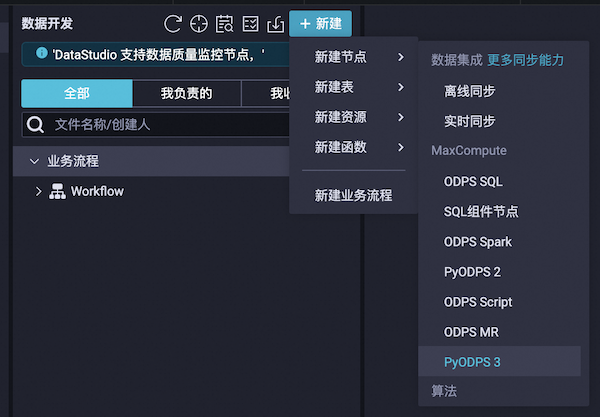# DataWorks 用户使用指南¶

## 新建工作流节点¶## ODPS入口¶

DataWorks 的 PyODPS 节点中，将会包含一个全局的变量 `odps` 或者 `o` ，即 ODPS 入口。用户不需要手动定义 ODPS 入口。

```print(o.exist_table('pyodps_iris'))
```

## 执行SQL¶

Dataworks 上默认没有打开 instance tunnel，即 instance.open_reader 默认走 result 接口（最多一万条）。 打开 instance tunnel，通过 reader.count 能取到记录数，如果要迭代获取全部数据，则需要关闭 limit 限制。

```options.tunnel.use_instance_tunnel = True
options.tunnel.limit_instance_tunnel = False  # 关闭 limit 读取全部数据

# 能通过 instance tunnel 读取全部数据
```

```with instance.open_reader(tunnel=True, limit=False) as reader:
# 这次 open_reader 会走 instance tunnel 接口，且能读取全部数据
```

## DataFrame¶

### 执行¶

```from odps.df import DataFrame

iris = DataFrame(o.get_table('pyodps_iris'))
for record in iris[iris.sepal_width < 3].execute():  # 调用立即执行的方法
# 处理每条record
```

```from odps import options
from odps.df import DataFrame

options.interactive = True  # 在开始打开开关

iris = DataFrame(o.get_table('pyodps_iris'))
print(iris.sepal_width.sum())  # 这里print的时候会立即执行
```

## 获取调度参数¶

```print('ds=' + args['ds'])
```
```ds=20161116
```

```o.get_table('table_name').get_partition('ds=' + args['ds'])
```

## 受限功能¶

• DataFrame的plot函数

DataFrame 自定义函数需要提交到 MaxCompute 执行。由于 Python 沙箱的原因，第三方库只支持所有的纯 Python 库以及 Numpy， 因此不能直接使用 Pandas，可参考 第三方库支持 上传和使用所需的库。DataWorks 中执行的非自定义函数代码可以使用平台预装的 Numpy 和 Pandas。其他带有二进制代码的三方包不被支持。